# 第四章（上）

The cv::Mat Class: N-Dimensional Dense Arrays
Mat n维稠密阵列
The cv::Mat class can be used for arrays of any number of dimensions. The data is                  mat可以作为任意维的数组。这些数据可以被认为以“光栅扫描”
stored in the array in what can be thought of as an n-dimensional analog of “raster                   的形式进行了保存。也就是说，在一维数据中，数据是连续的。
scan order.” This means that in a one-dimensional array, the elements are sequential.
In  a  two-dimensional  array,  the  data  is  organized  into  rows,  and  each  row  appears       在二维数据中，数据分割成行，而且每一个行紧密排列。
one after the other. For three-dimensional arrays, each plane is filled out row by row,               在三维数据中，每一个平面连续排列。
and then the planes are packed one after the other.

Each matrix contains a flags element signaling the contents of the array, a dims ele‐                   每一个矩阵包含一个flag，分别表明不同特性。dims 表明维度；rows cols表明 rows
ment  indicating  the  number  of  dimensions,  rows  and  cols  elements  indicating  the            columns大小，此外还包含一个指向数据保存位置的指针；还有一个ptr<>使用频率
number  of  rows  and  columns  (these  are  not  valid  for  dims>2),  a  data  pointer  to          计数。ptr<>可以是Mat使用起来非常类似smart 的指针。
where the array data is stored, and a refcount reference counter analogous to the ref‐
erence counter used by cv::Ptr<>. This latter member allows cv::Mat to behave very
much like a smart pointer for the data contained in data. The memory layout in data                 Mat的内存布局很大程度上由step[]来表示。内存结构为
is described by the array step[]. The data array is laid out such that the address of an
element whose indices are given by (i0, ii,  … , iN） is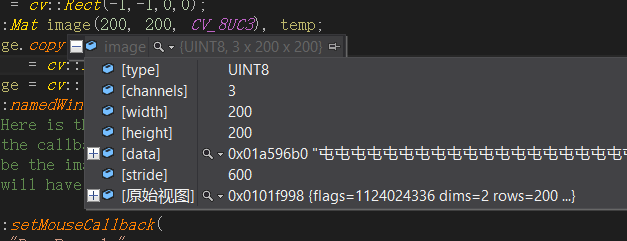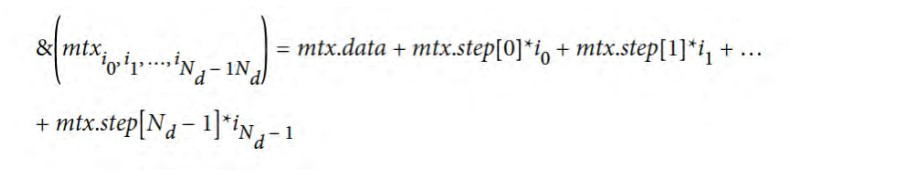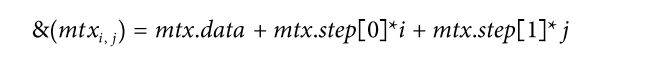Mat的多种定义方法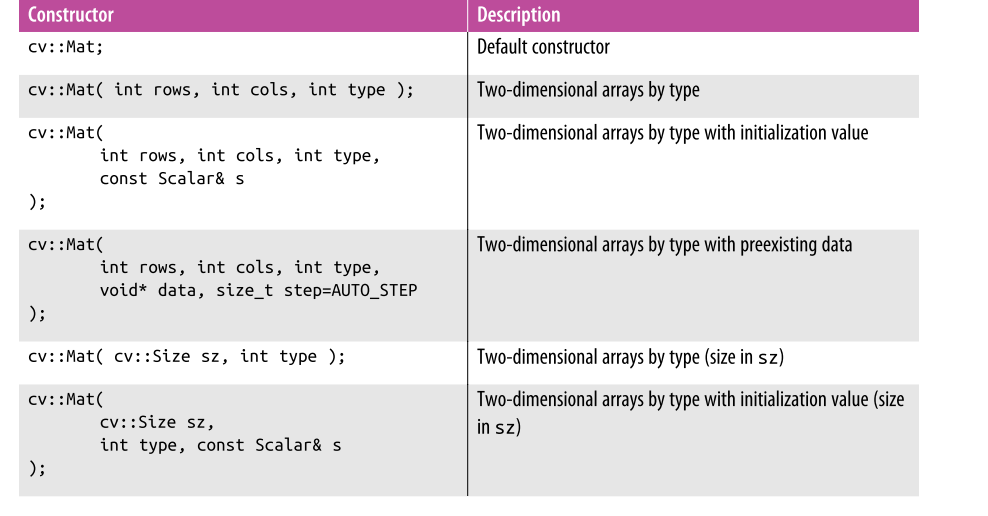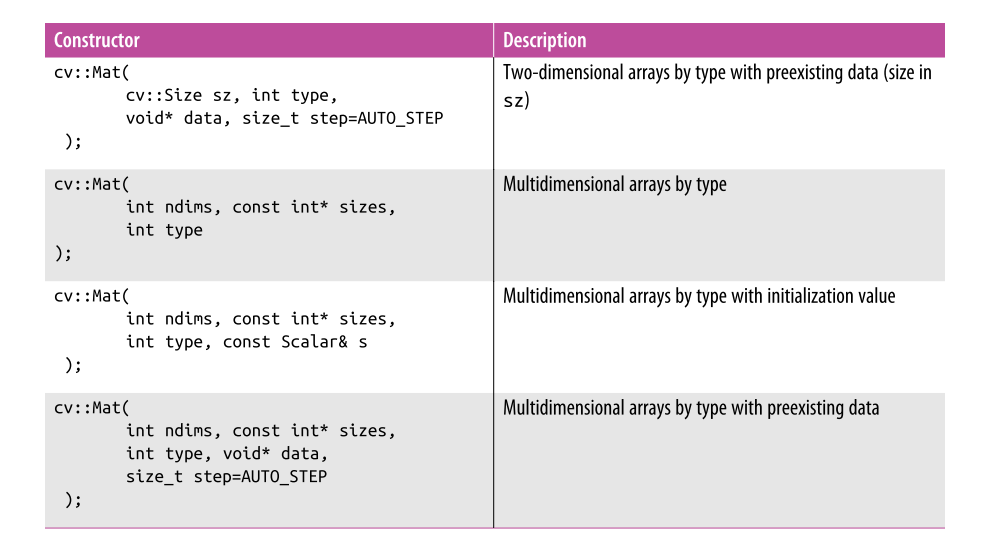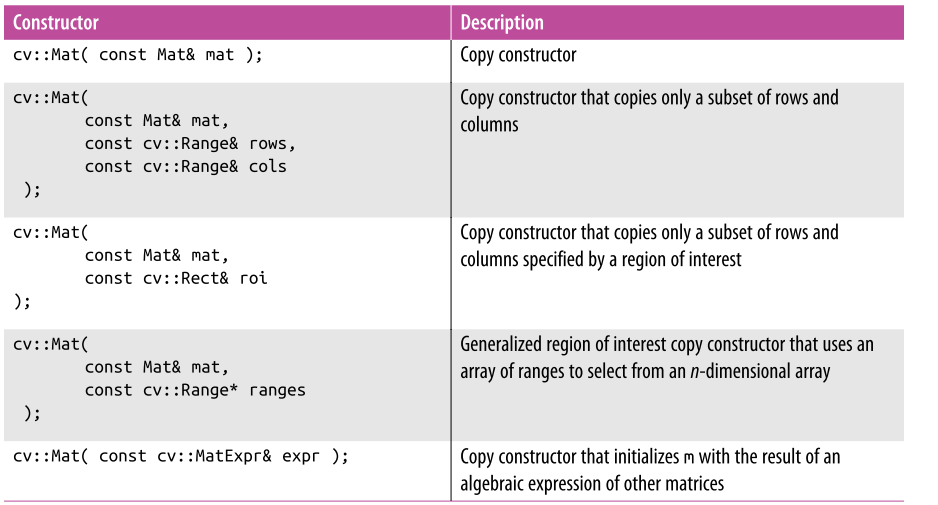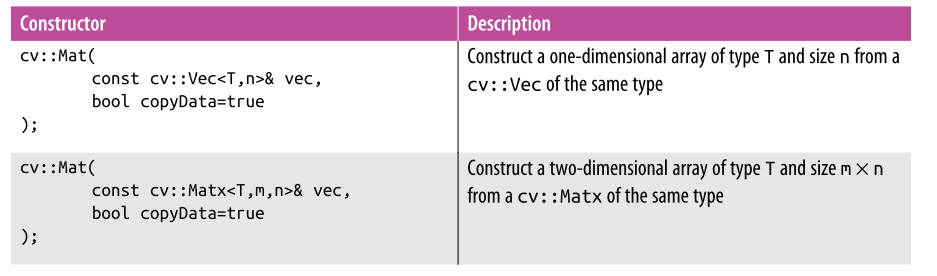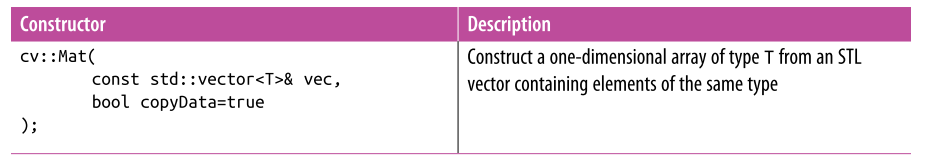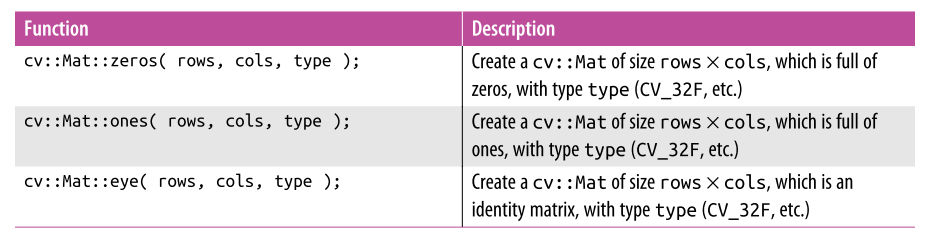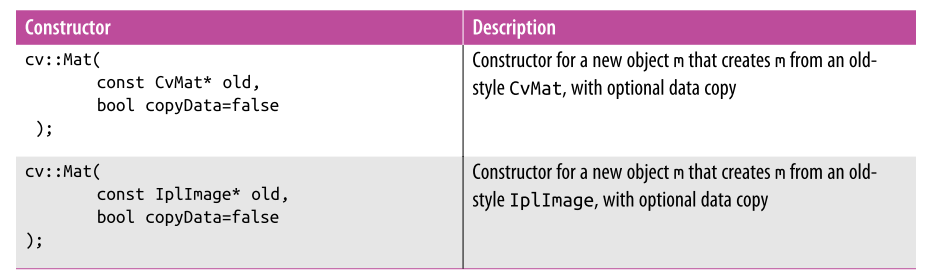### 一、at<typename>(i,j)

Mat类提供了一个at的方法用于取得图像上的点，它是一个模板函数，可以取到任何类型的图像上的点。下面我们通过一个图像处理中的实际来说明它的用法。

 void colorReduce(Mat& image,int div){    for(int i=0;i<image.rows;i++){        for(int j=0;j<image.cols;j++){            image.at<Vec3b>(i,j)=image.at<Vec3b>(i,j)/div*div+div/2;             image.at<Vec3b>(i,j)=image.at<Vec3b>(i,j)/div*div+div/2;             image.at<Vec3b>(i,j)=image.at<Vec3b>(i,j)/div*div+div/2;        }    }}

image.at<uchar>(i,j)：取出灰度图像中i行j列的点。

image.at<Vec3b>(i,j)[k]：取出彩色图像中i行j列第k通道的颜色点。其中uchar,Vec3b都是图像像素值的类型，不要对Vec3b这种类型感觉害怕，其实在core里它是通过typedef Vec<T,N>来定义的，N代表元素的个数，T代表类型。

### 二、高效一点：用指针来遍历图像

 void colorReduce(const Mat& image,Mat& outImage,int div){    // 创建与原图像等尺寸的图像    outImage.create(image.size(),image.type());    int nr=image.rows;    // 将3通道转换为1通道    int nl=image.cols*image.channels();   for(int k=0;k<nr;k++)    {        // 每一行图像的指针        const uchar* inData=image.ptr<uchar>(k);        uchar* outData=outImage.ptr<uchar>(k);        for(int i=0;i<nl;i++)        {            outData[i]=inData[i]/div*div+div/2;        }    }}

### 三、更高效的方法

 void colorReduce(const Mat& image,Mat& outImage,int div){    int nr=image.rows;      int nc=image.cols;       outImage.create(image.size(),image.type());       if(image.isContinuous()&&outImage.isContinuous())    {        nr=1;        nc=nc*image.rows*image.channels();    }        for(int i=0;i<nr;i++)    {              const uchar* inData=image.ptr<uchar>(i);             uchar* outData=outImage.ptr<uchar>(i);           for(int j=0;j<nc;j++)        {            *outData++=*inData++/div*div+div/2;        }    }}

image.step返回图像一行像素元素的个数（包括空白元素），image.elemSize()返回一个图像像素的大小。

&image.at<uchar>(i,j)=image.data+i*image.step+j*image.elemSize();

### 四、还有吗？用迭代器来遍历。

MatIterator_<Vec3b> it;Mat_<Vec3b>::iterator it;

MatConstIterator<Vec3b> it;  Mat_<Vec3b>::const_iterator it;

 
 void colorReduce(const Mat& image,Mat& outImage,int div){   
 
 outImage.create(image.size(),image.type());
 
 MatConstIterator_<Vec3b> it_in=image.begin<Vec3b>();
 
 MatConstIterator_<Vec3b> itend_in=image.end<Vec3b>();
 
 MatIterator_<Vec3b> it_out=outImage.begin<Vec3b>();
 
 MatIterator_<Vec3b> itend_out=outImage.end<Vec3b>();
 
 while(it_in!=itend_in)    {        (*it_out)=(*it_in)/div*div+div/2;        (*it_out)=(*it_in)/div*div+div/2;        (*it_out)=(*it_in)/div*div+div/2;        it_in++;        it_out++;    }
 
}
 

tips:在opencv中，两种生成随机数的方法

randu(src,-1.0f,1.0f);

rng.fill(src,RNG::UNIFORM,0.f,1.f);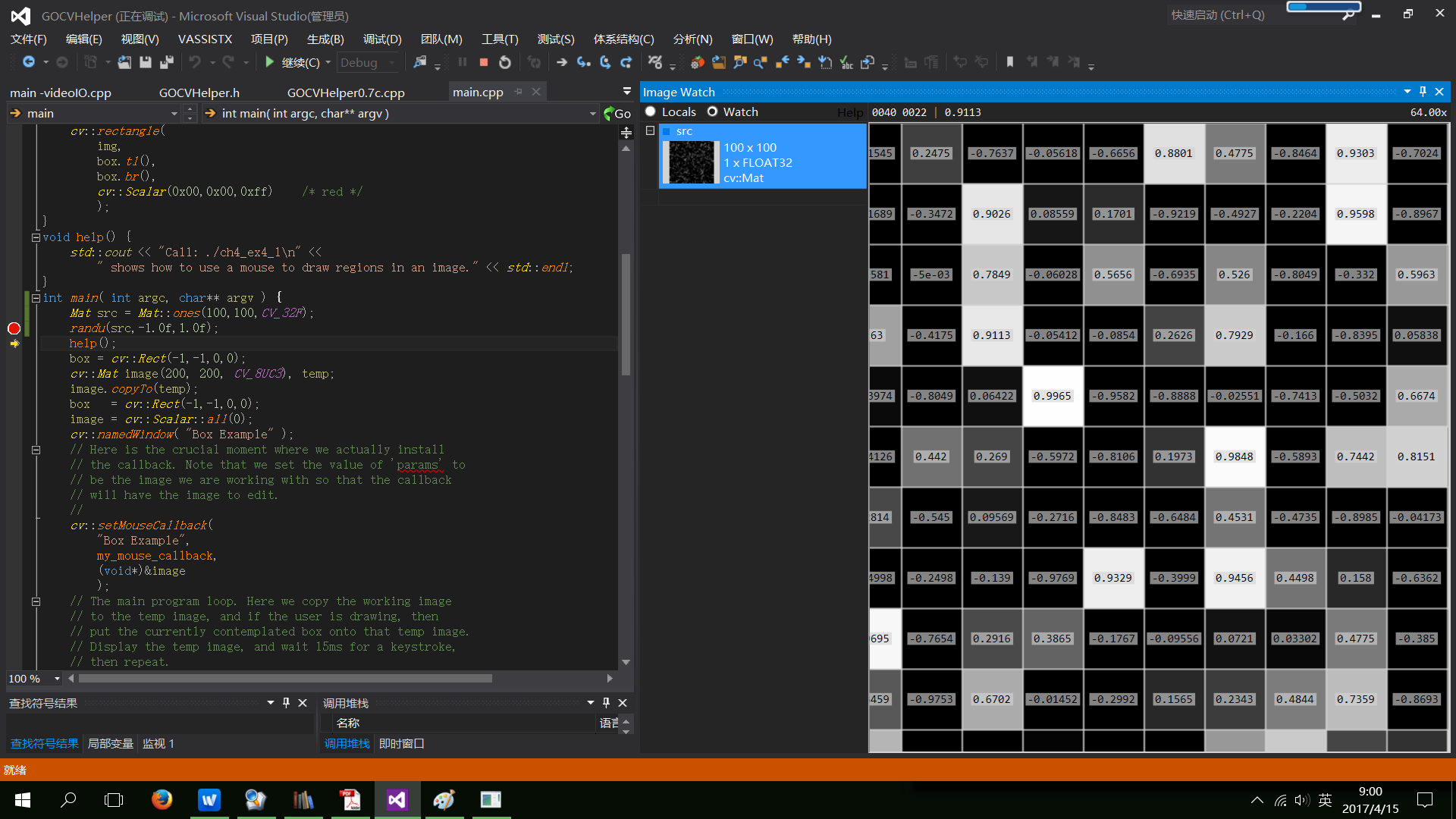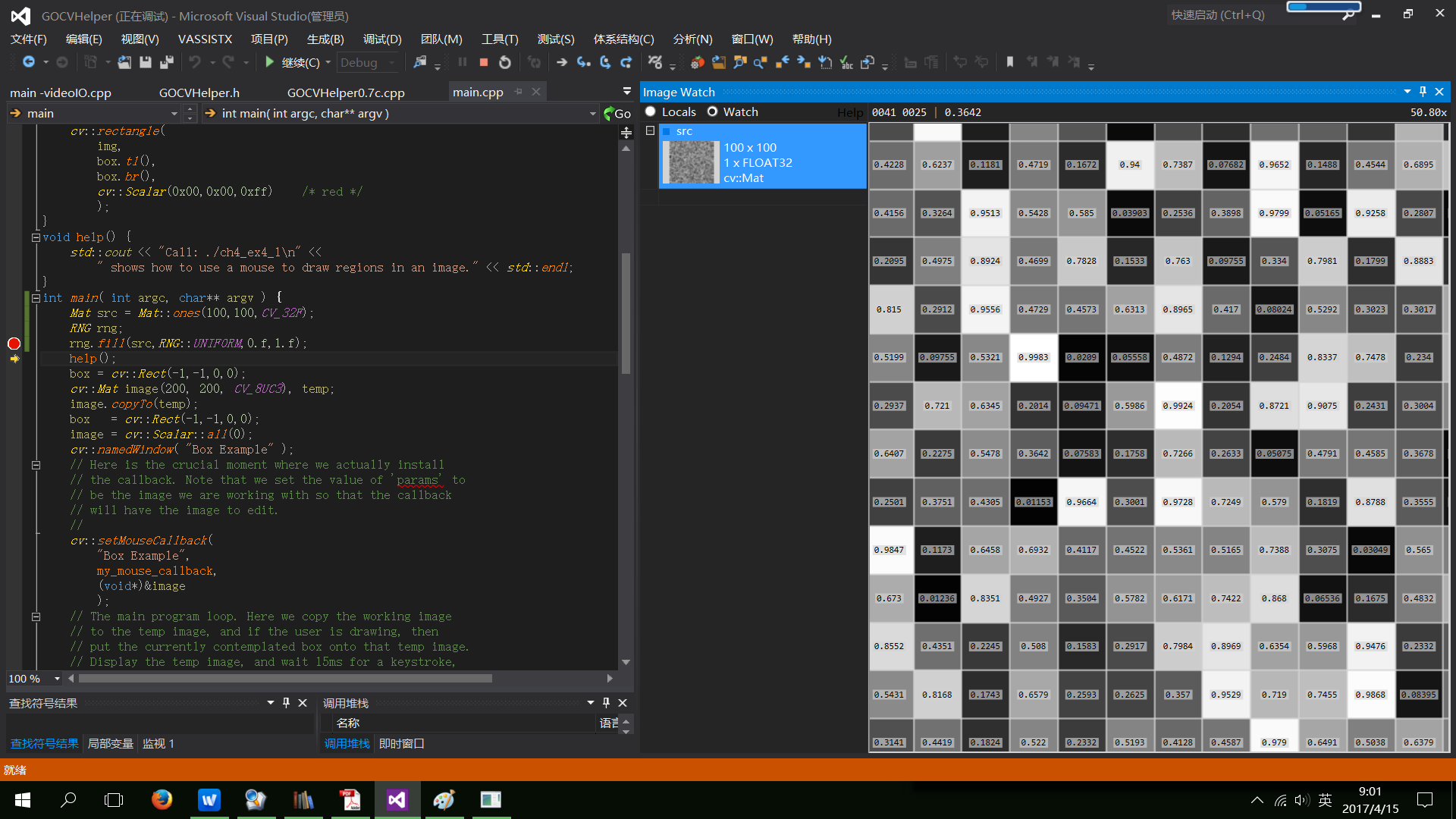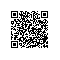使用钉钉扫一扫加入圈子
+ 订阅